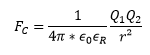Are you more of a visual learner? Check out our online video lectures and start your physics course now for free!Image: “A new instrument called GRAVITY has been shipped to Chile and successfully assembled and tested at the Paranal Observatory. GRAVITY is a second generation instrument for the VLT Interferometer and will allow the measurement of the positions and motions of astronomical objects on scales far smaller than is currently possible. The picture shows the instrument under test at the Paranal Observatory in July 2015.” By MPE/GRAVITY team. License: CC BY 4.0

## Force and Newton’s Laws

First law: the law of inertia
An object’s velocity will not change unless acted on by an external net force.

Second law: the basic equation of mechanics
The sum of forces on an object will be equal to its mass times its acceleration: F = m a.

Third law: the law of action-reaction
For every force, there is a reaction force equal in magnitude and opposite in direction.

## Important Forces

Forces deform objects, set them into motion, or accelerate their motion. Force is an interaction between objects that changes the energy of an object. The general equation for force is the following:

F = m a

F ⇒ force (N)
m ⇒ mass (kg)

a ⇒ acceleration (m/s2)

Different types of forces are displayed in the equations below:

 Force Equation Description Force of gravity (weight) FG FG = m g FG is the force with which the earth attracts another object towards itself (‘downward’). Earth’s acceleration (gravity) is a constant quantity: g = 9.81 m/s2. Buoyancy (upthrust) FA FA = ρFl g V FA = FG FA < FG FA > FG FA is the upward force in fluids. ρFl = density of the fluid (kg/m³) V = volume of the object placed in the fluid (m³) Spring force FD FD = D ΔL FD describes the deflection of a spring. D = spring constant (material-specific constant) (N/m) ∆L = the length/stretch of a spring (m) Normal force FN FN = cosα FG FN is the force exerted perpendicular to the surface of an object. When exerted horizontally, normal force equals weight. α = angle of the inclined surface measured from the horizontal Tangential force FT FT = sinα FG FT is the force exerted parallel to the surface of an object. Static friction force FH FH = μH FN FH  is the force exerted by a surface as an object moves across it. It depends on the material and surface quality of the interacting bodies. μH = static friction coefficient, without unit Kinetic (sliding) friction Force FGl FGl = μG FN FGl is the force that occurs when 2 objects are moving relative to each other and rub together. μG = kinetic friction coefficient, without unit Rolling resistance FRoll FRoll = μRoll FN FRoll is the force resisting the motion when a body rolls on a surface. μRoll = rolling resistance coefficient, without unit Lubricants decrease the rolling resistance coefficient, and thus, less force is required when moving 2 colliding bodies. Centrifugal force FZf FZf = m ω r FZf is the inertial force that causes an object in a rotating reference frame to move outward, away from the axis. r = radius of the circle (m) m = mass of the object (kg) ω = angular velocity (1/s) Centripetal force FZP FZP = – FZf FZP is a force that is directed from the radius toward the center of the circle. It is opposite of the centrifugal force. Coulomb force FCFC describes how strongly 2 objects or particles are attracted to each other. It depends on the charge of the objects/particles and the distance between them. ε0 = electric field constant εr = dielectric constant Q1, Q2 = charge of the 2 objects (C) r = distance of the 2 objects to the center (m)## Uniform Circular Motion

In this type of motion, an object is moving along a circular path. Since the velocity is a vector, its constantly changing directions balance each other out. Thus, uniform circular motion is defined by the constant sum of velocity or to put it simply, if a car is driving in a circle with the speed of 50 km/h, the acceleration is constant, yet the direction constantly changes.ω ⇒ angular velocity (1/s)
α ⇒ angular acceleration (1/s2)
n ⇒ rotational speed (1/s)
π ⇒ Pi (approx. 3.14)

## Center of Mass

The geometric center often differs from the center of mass, since the latter depends on the density (and therefore, the mass) of an object. It can be defined as the centroid of a system with any number of points of the same mass A0, A1, A2… An.MS = Center of mass, no unitImage: Center of gravity. By Lecturio

The entire weight of an object acts at its center of mass (also called centroid). The center of the mass of a human body in a standing posture, for example, lies in the hip area. However, the center of mass can change depending on the posture and motion, and in case of extreme movements, it can even be located outside the body.

The posture of a body determines the type of equilibrium. We distinguish the following types:

• Stable equilibrium: The body comes from the deflected state back to the original posture.
• Unstable equilibrium: After coming back from a deflected state, the body, which had been in equilibrium before, moves further away from the equilibrium state.
• Neutral equilibrium: The body takes a new weight.(Votes: 2, average: 3.50)Loading...